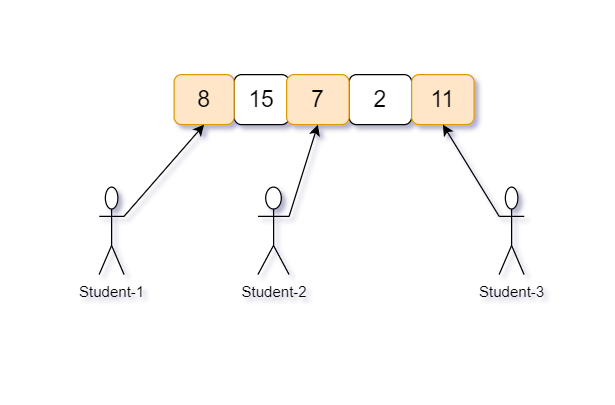New update is available. Click here to update.

# Chocolate Problem

Contributed by
Ayush Thakur
Last Updated: 23 Feb, 2023
Medium0/80
Avg time to solve 15 mins
Success Rate 85 %Share16 upvotes

## Problem Statement

#### Example :

``````Given 'N' : 5 (number of packets) and 'M' : 3 (number of students)
````````````And chocolates in each packet is : {8, 11, 7, 15, 2}

All possible way to distribute 5 packets of chocolates among 3 students are -

( 8,15, 7 ) difference of maximum-minimum is ‘15 - 7’ = ‘8’
( 8, 15, 2 ) difference of maximum-minimum is ‘15 - 2’ = ‘13’
( 8, 15, 11 ) difference of maximum-minimum is ‘15 - 8’ = ‘7’
( 8, 7, 2 ) difference of maximum-minimum is ‘8 - 2’ = ‘6’
( 8, 7, 11 ) difference of maximum-minimum is ‘11 - 7’ = ‘4’
( 8, 2, 11 ) difference of maximum-minimum is ‘11 - 2’ = ‘9’
( 15, 7, 2 ) difference of maximum-minimum is ‘15 - 2’ = 13’
( 15, 7, 11 ) difference of maximum-minimum is ‘15 - 7’ = ‘8’
( 15, 2, 11 ) difference of maximum-minimum is ‘15 - 2’ = ‘13’
( 7, 2, 11 ) difference of maximum-minimum is ‘11 - 2’ = ‘9’

Hence there are 10 possible ways to distribute ‘5’ packets of chocolate among the ‘3’ students and difference of combination (8, 7, 11) is ‘maximum - minimum’ = ‘11 - 7’ = ‘4’ is minimum in all of the above.
``````
Detailed explanation ( Input/output format, Notes, Images )##### Constraints:
``````1 <= T <= 50
2 <= M <= N <= 10^4
1 <= CHOCOLATES[i] <= 10^9

Time Limit : 1 sec
``````
##### Sample Input 1 :
``````2
3 2
7 2 4
4 3
3 5 1 6
``````
##### Sample Output 1 :
``````2
3
``````
##### Explanation For Sample Input 1 :
``````Test Case 1 :

All possible way to distribute 3 packets of chocolate among 2 students are -

( 7, 2 ) difference is ‘7 - 2’ = ‘5’
( 7, 4 ) difference is ‘7 - 4’ = ‘3’
( 2, 4 ) difference is ‘4 - 2’ = ‘2’

There are three ways to distribute 3 packets of chocolate among 2 students but pair ( 4, 2 ) has minimum difference in ‘maximum - minimum’ = ‘4 - 2’ = ‘2’

Test Case 2 :

All possible way to distribute 4 packets of chocolate among 3 students are -

( 3, 5, 1 )  difference is ‘5 - 1’ = ‘4’
( 5, 1, 6 )  difference is ‘6 - 1’ = ‘5’
( 1, 6, 3 )  difference is ‘6 - 1’ = ‘5’
( 6, 5, 3 )  difference is  ‘6 - 3’ = ‘3’

There are four options to choose 3 packets of chocolate. Only ( 6, 5, 3 ) pair has the minimum difference ‘6 - 3’ = ‘3’ comparing other pair of difference ( 4, 5, 5 )
``````
##### Sample Input 2 :
``````2
7 3
7 3 2 4 9 12 56
8 5
3 4 1 9 56 7 9 12
``````
##### Sample Output 2 :
``````2
6
``````AutoConsole